×#### Thank you for registering.

One of our academic counsellors will contact you within 1 working day.

Click to Chat

1800-1023-196

+91-120-4616500

CART 0

• 0

MY CART (5)

Use Coupon: CART20 and get 20% off on all online Study Material

ITEM
DETAILS
MRP
DISCOUNT
FINAL PRICE
Total Price: Rs.

There are no items in this cart.
Continue Shopping```Basic Concepts of Indefinite Integrals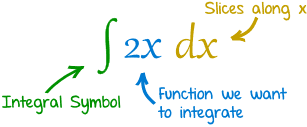Let F(x) be a differentiable function of x such that d/dx [F(x)] = f(x). Then F(x) is called the integral of f(x). Symbolically, it is written as ∫ f(x) dx = F(x).

f(x), the function to be integrated, is called the integrand.

F(x) is also called the anti-derivative (or primitive function) of f(x).

As the differential coefficient of a constant is zero, we have

d/dx (F(x)) = f(x) ⇒ d/dx [F(x) + c] = f(x).

Therefore, ∫ f(x) dx = F(x) + c. This constant c is called the constant of integration and can take any real value.Integration is the reverse process of differentiation.

Two integrals of the same function can differ only by a constant.

If integral of a function ‘A’ is ‘B’, then the differential of function ‘B’ is ‘A’.

The indefinite integral of any function always has a constant.

If integrand f(x) cab be written as the product of two functions f1(x) and f2(x) where f2(x) is a function of integral of f1(x), then put integral of f1(x) = t.

Basic Formulae

Some of the important integrals of some frequently used functions are listed below: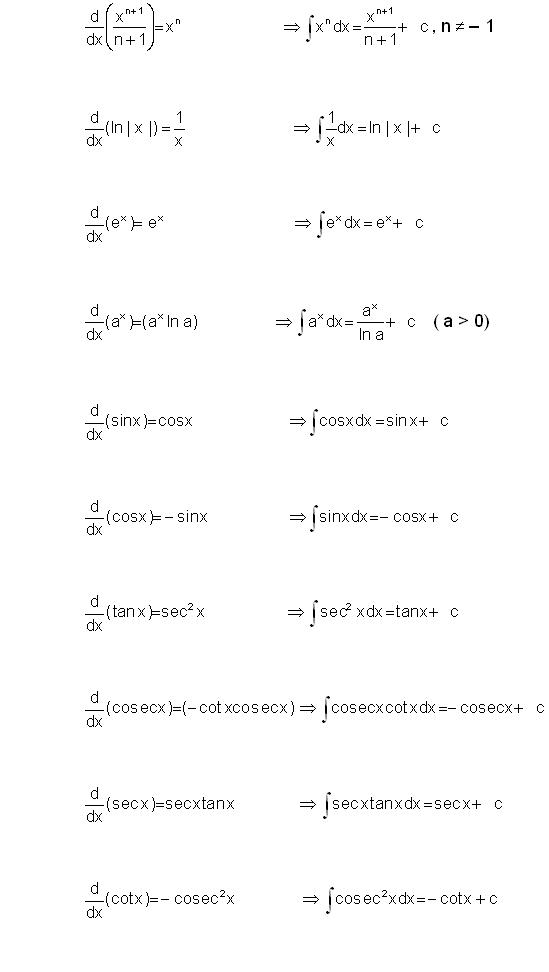Some Standard Formulae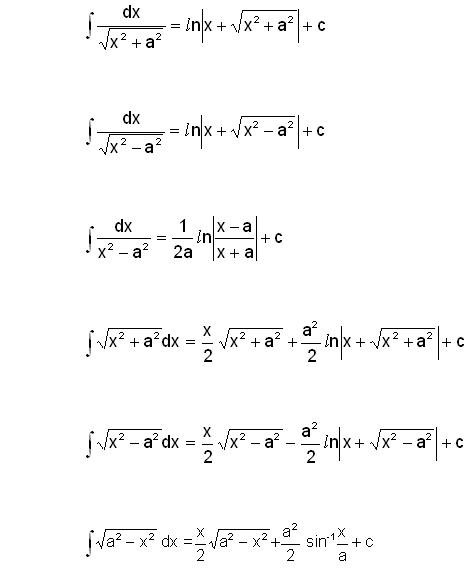Watch this Video for more reference

Example 1:

Evaluate ∫ 1/(1 + sin x) dx.

Solution:

In order to compute this integral, we multiply and divide it by (1-sin x) and so we have,

∫ 1/(1+ sin x) . (1 – sin x)/(1 - sin x) dx

= ∫ (1 – sin x)/(1 - sin2x) dx

= ∫ [(1 – sin x)/cos2x] dx

Now splitting it into two terms,

= ∫(1/cos2x dx – ∫ (sin x /cos2x) dx

=  ∫ sec2x dx – ∫ tan x sec x dx

= tan x – sec x + c.

________________________________________________________________________________

Example 2:

Evaluate ∫ sin x /(1 + sin x) dx.

Solution:

∫ sin x /(1 + sin x) dx

= ∫ (sin x + 1 – 1)/(1 + sin x) dx

= ∫1 –  ∫1/(1 + sin x) dx

= ∫1 – ∫(1 – sin x)/(1–sin2 x) dx

= ∫1 dx –  ∫(1–sin x)/cos2 x dx

= ∫(1 – sec2 x + sec x tan x) dx

= x - tan x - sec x + C.

_____________________________________________________________________________

Example 3:

Evaluate ∫(sin3x + cos3x)/(sin2x cos2x) dx.

Solution: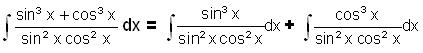= ∫ tan x sec x dx + ∫ cot x cosec x dx

= sec x – cosec x + c.

Methods of Integration

The following chart illustrates the various methods of integration: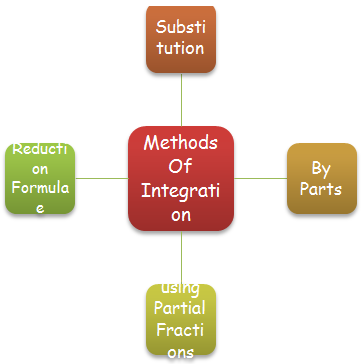These methods have been explained in detail in the coming sections. Students are advised to refer the coming sections to understand the concepts. Integration by substitution can further be divided into three parts: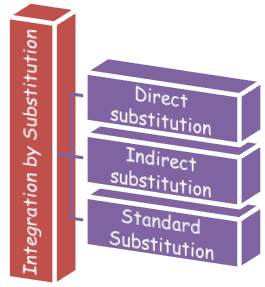It is better to memorize some of the standard substitutions as they often prove helpful in solving tricky questions.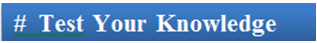Q1. Integration is the inverse process of

(a) the coefficient of x2.

(b) anti differentiation

(c) differentiation

(d) anti derivative

Q2. The answer of an indefinite integral

(a) necessarily has a constant

(b) may or may not have a constant

(c) cannot have a constant

(d) none of these

Q3. The differential coefficient of a constant is

(a) number itself

(b) zero

(c) constant

(d) none of these

Q4. If ∫ f(x) dx = F(x) + c, then

(a) F(x) is called the primitive function of f(x).

(b) f(x) is called the primitive function of F(x).

(c) F(x) is called the derivative function of f(x).

(d) f(x) is called the root of F(x).

Q5. While solving integrals of the form x2 + a2 or √(x2 + a2), the substitution used is

(a) x = a sec θ

(b) x = a tan θ

(c) x = a cosec θ

(d) x = a sin θQ1.

Q2.

Q3.

Q4.

Q5.

(c)

(a)

(b)

(a)

(b)

Related Resources

You may wish to refer Integration by Substitution.

For getting an idea of the type of questions asked, refer the previous year papers.

To read more, Buy study materials of Indefinite integral comprising study notes, revision notes, video lectures, previous year solved questions etc. Also browse for more study materials on Mathematics here.
```### Course Features

• 731 Video Lectures
• Revision Notes
• Previous Year Papers
• Mind Map
• Study Planner
• NCERT Solutions
• Discussion Forum
• Test paper with Video Solution